# Problems on Trains

#### Video Lesson on Problems on Trains

Problems on trains are very common in competitive exams.

Various types of questions are asked on trains. Questions on trains are solved using the concept of time, speed and distance i.e. we use the formulas of time, speed and distance to solve questions on trains.

While studying the chapter “Trains”, we are required to deal with following scenarios:

1. Two trains moving in opposite direction.
2. Two trains moving in same direction.
3. A train crossing a stationary object of a given length like a platform or bridge.
4. A train crossing a stationary object like a pole or a man which can be considered as a point object.

### Important Points to Remember

1. If the length of one train is P and the length of second train is Q, the total distance to be covered is (P+Q)
2. 2) Finding Relative speed: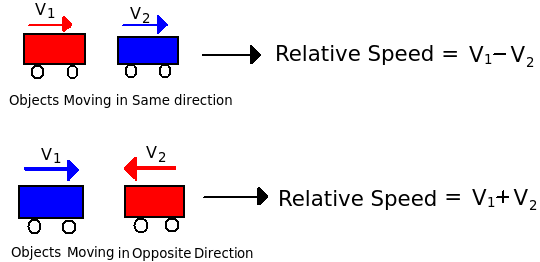3. If two trains of lengths P and Q move in opposite directions at V1 m/s and V2 m/s, then time taken by the trains to cross each other, can be calculated by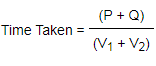4. If two trains of different lengths P and Q move in same direction at V1 m/s and V2 m/s, then time taken by the trains to cross each other, is calculated by

#### Question 1: A train travelling at 60 kmph crosses a man in 6 seconds. What is the length of the train?

Solution: Speed in m/sec = 60 × (5/18) = 50/3 m/sec

Time taken to cross the man = 6 secs

Therefore, Distance = (50/3)* 6 = 100 metres (i.e. the length of the train)

#### Question 2: A train travelling at 60 kmph crosses another train travelling in the same direction at 50 kmph in 30 seconds. What is the combined length of both the trains?

Solution: Speed of train A = 60 kmph = 60 × (5/18) = 50/3 m/sec

Speed of train B = = 50 kmph = 50 × (5/18) = 125/9 m/sec

The relative speed =(50/3) - (125/9) = 25/9 m/s (we have subtracted the two values because both the trains are going in the same direction)

Time taken by train A to cross train B = 30 secs

Distance = Speed × Time

Distance =25/9 × 30 = 250/3 metres (i.e. the combined length of both trains)### Quick Tips and Tricks

1. Time taken by a train of length L meter to pass a signal post or standing man = Time taken by the train to cover L meter.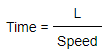A signal post or a standing man is considered to be the point object.
2. The time taken by a train of length L1 meter to pass a stationary object of length L2 is basically the time taken by the train to cover (L1 + L2) meter.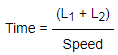3. The time taken by a train of length L1 meter to pass a moving object of length L2 is determined by considering the relative speed between the moving objects.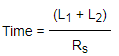Rs is the relative speed between moving objects in same or opposite direction.
L1 is the length of train.
L2 is the length of moving object other than train.
4. Two trains start from two points P and Q at the same time and move towards each other. These trains take p and q seconds to reach points Q and P respectively, the relation between them is given by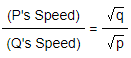### Conversion of Units

1. To convert km/hr into m/s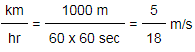Example: 50 km/hr = 50 x 5 /18 = 13.88 m/s
2. To convert m/s into km/hr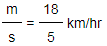Example: 50 m/s = 50 x 18 / 5 = 180 km/hr
3. To convert minutes into seconds, multiply by 60
4. To convert hours into seconds, multiply by 60 × 60
Note:The most important thing to remember in this chapter is to ensure the right usage of units and convert them wherever required.

#### Question 8: How long will a 150 m long train running at a speed of 60 kmph take to cross a bridge of 300 m?

Solution: Total Distance = 300 + 150 = 450 m

Speed = 60 kmph = 60 × (5/18)=(50/3) m/sec

Distance = Speed × Time

450 =(50/3) × Time

Time = 27 seconds

### More Educational and Fun StuffMore In-depth knowledge about what you need. Detailed about test preparation, English Writing, TOEFL, and IELTS.